# Labyrinth Chaos

J. C. Sprott
Department of Physics, University of Wisconsin, 1150 University Ave., Madison, WI 53706, USA

Konstantinos E. Chlouverakis
Department of Informatics and Telecommunications, University of Athens, Athens 15784, Greece

(Received July 24, 2006; Revised August 29, 2006)

### ABSTRACT

A particularly simple and mathematically elegant example of chaos in a three-dimensional flow is examined in detail. It has the property of cyclic symmetry with respect to interchange of the three orthogonal axes, a single bifurcation parameter that governs the damping and the attractor dimension over most of the range 2 to 3 (as well as 0 and 1) and whose limiting value b = 0 gives Hamiltonian chaos, three-dimensional deterministic fractional Brownian motion, and an interesting symbolic dynamic.

Ref: J. C. Sprott and K. E. Chlouverakis, International Journal of Bifurcation and Chaos 17, 2097-2108 (2007)

The complete paper is available in PDF format.

The BASIC computer code used to produce the figures is available.

Fig. 1. Kaplan-Yorke dimension and Lyapunov exponents versus b showing the route to chaos.Fig. 2. Bifurcation diagram (local maximum of x) and Lyapunov exponents versus b showing the route to chaos in greater detail.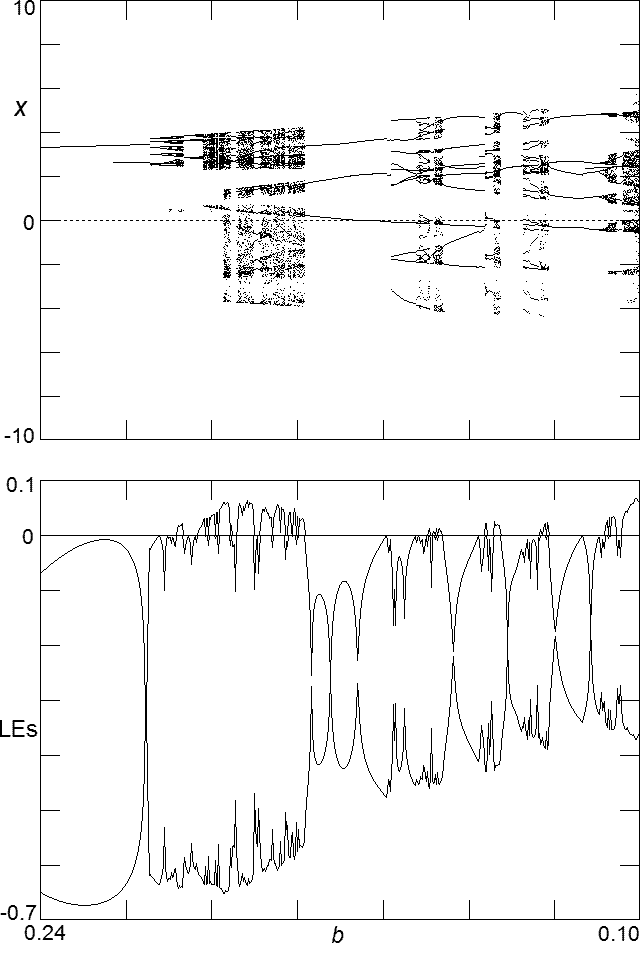Fig. 3. Kaplan-Yorke dimension and Lyapunov exponents versus b showing the route to chaos. In the upper plot, the circles with error bars are values of the correlation dimension.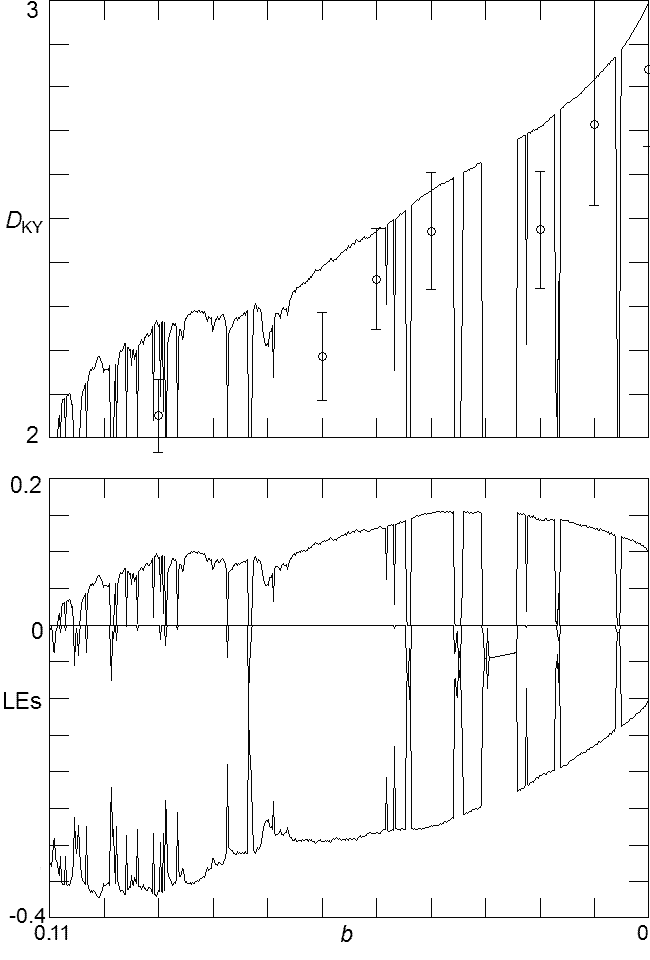Fig. 4. Cross-section of the attractor in yz-space at x = 0 for four values of b. The axes are -20 to 20 for each case.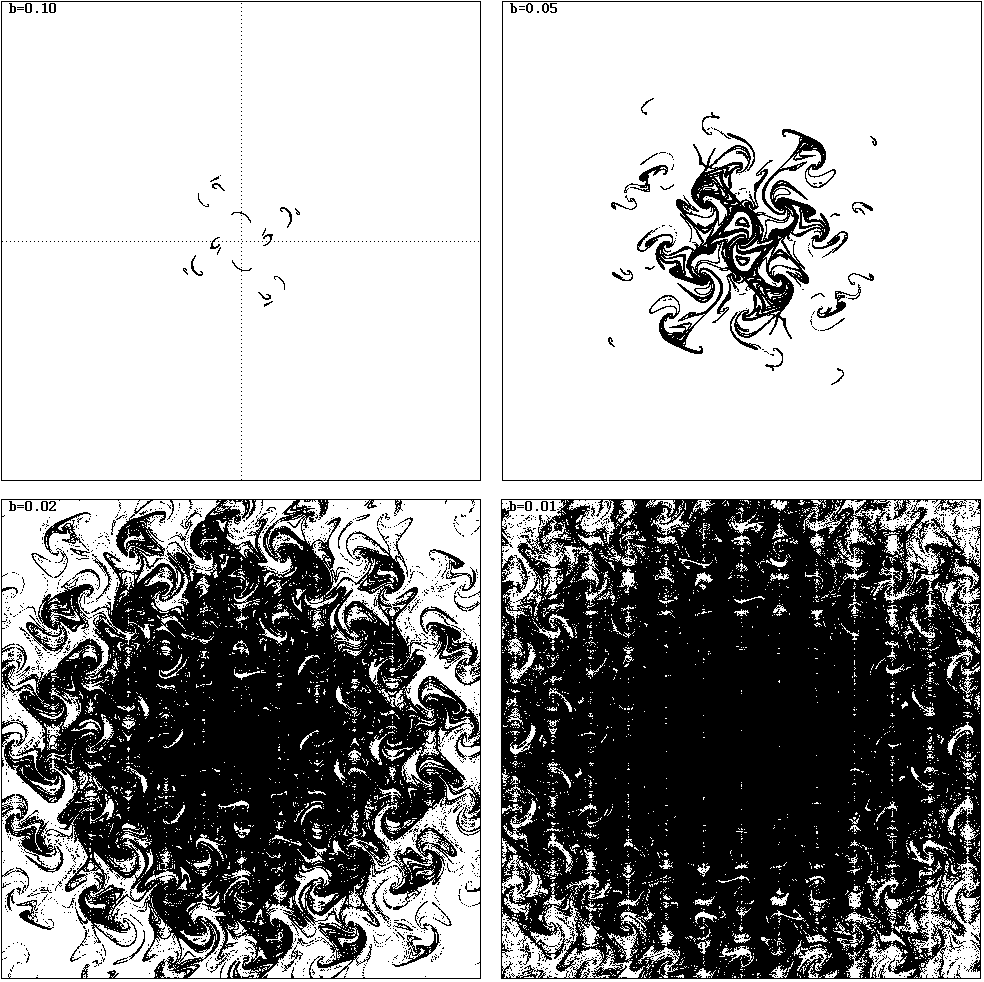Fig. 5. Standard deviation and kurtosis for the excursion of the trajectory from the origin for the attractors as a function of b.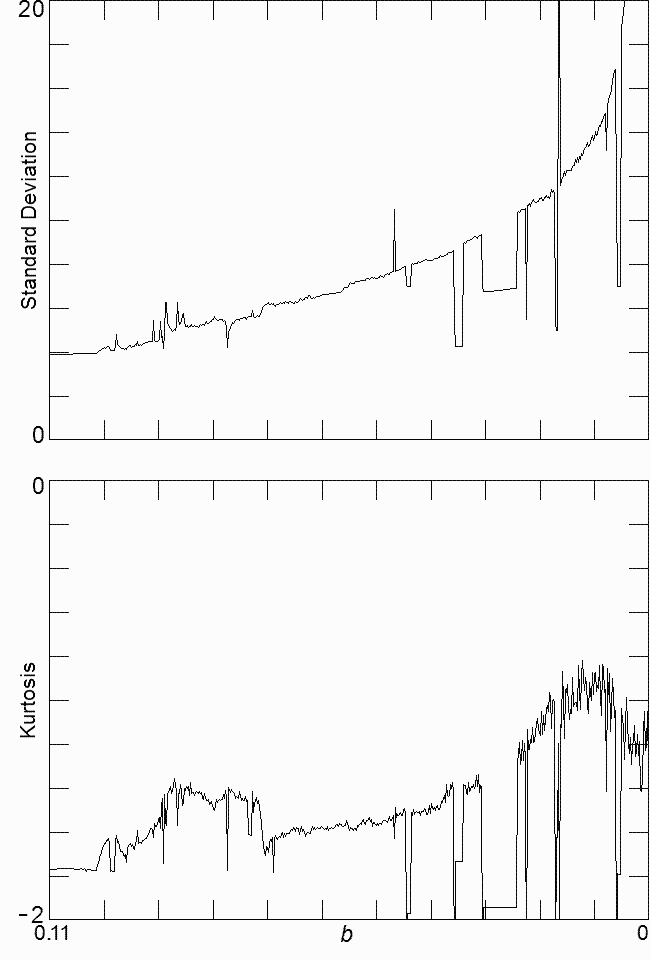Fig. 6. Plot showing regions of multiple coexisting attractors as a function of b.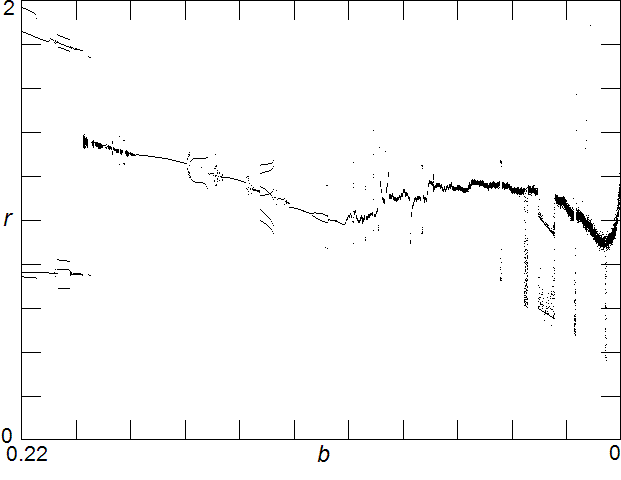Fig. 7. Multiple coexisting attractors.

Fig. 8. Six coexisting strange attractors at b = 0.203.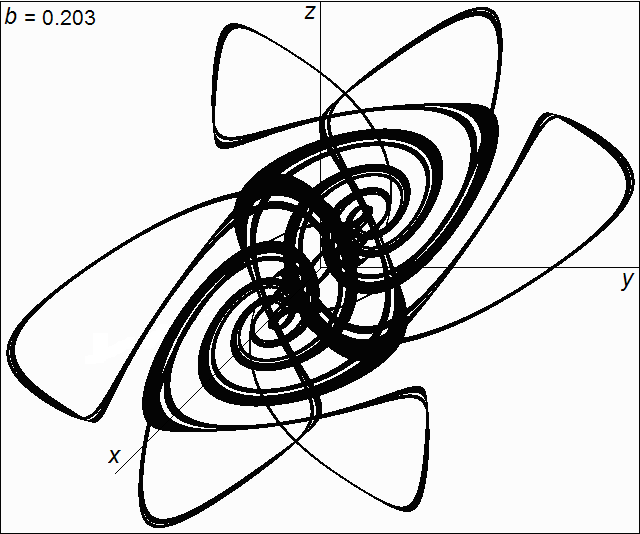Fig. 9. Cross-section of the chaotic sea at (x  mod 2pi) = 0 for the conservative case with b = 0.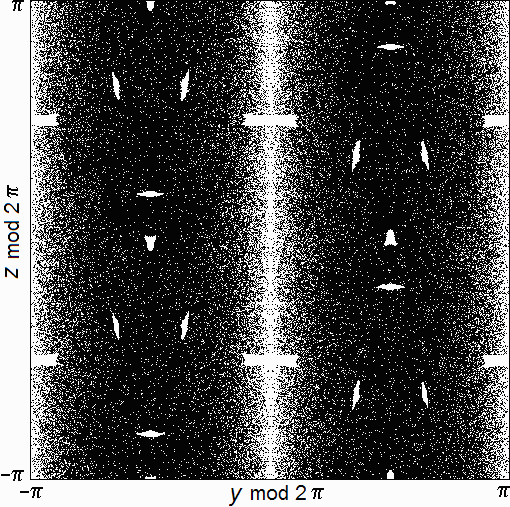Fig. 10. Stereogram showing the regions where quasiperiodic trajectories occur for b = 0. The view is looking down along the x-axis, and the different colors denote the six directions in which trajectories drift.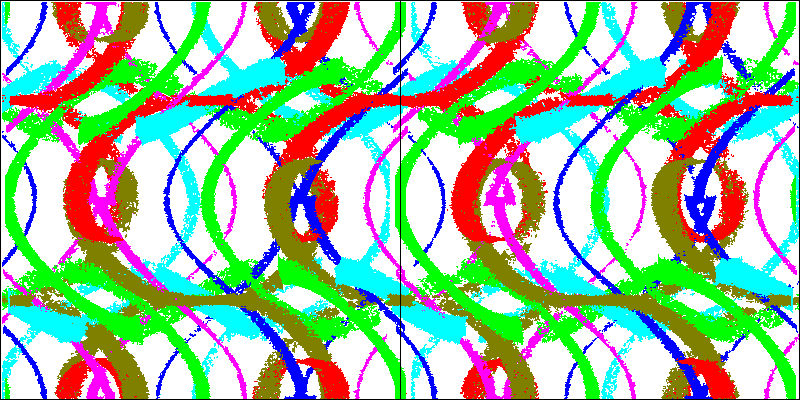Fig. 11. Cross-section at (x mod 2pi) = 0 showing the quasiperiodic orbits for b = 0 surrounded by a KAM surface.Fig. 12. Brownian motion of a trajectory in the chaotic sea (black) along with a quasiperiodic trajectory (red).Fig. 13. Probability distribution function of x for 5 × 106 initial conditions near the origin after a time of 4 × 103. The red curve is a Gaussian distribution with the same standard deviation and area.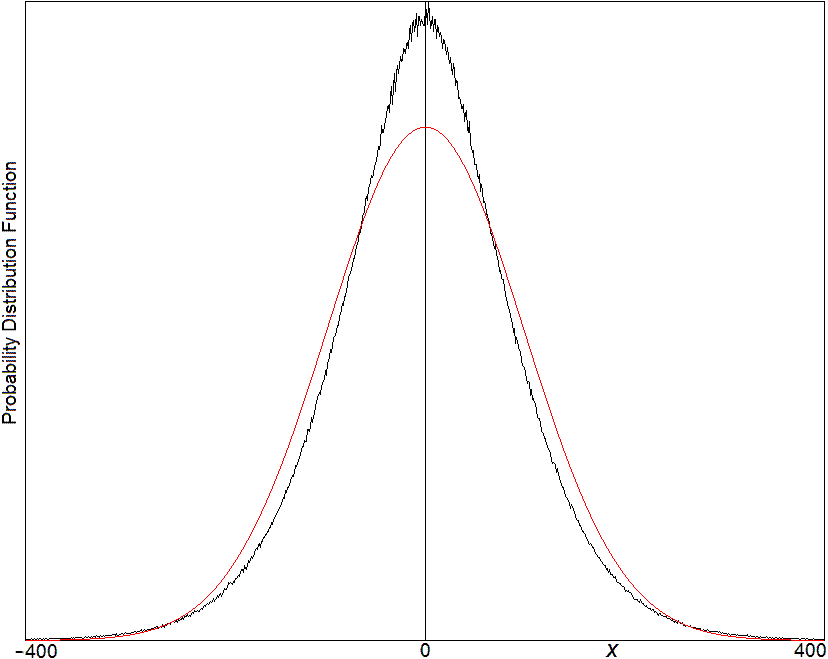Fig 14. Projection of the trajectory onto the x-axis showing an example of intermittency where the trajectory approaches the quasiperiodic region with initial conditions (0.05, 0.09, 0.05).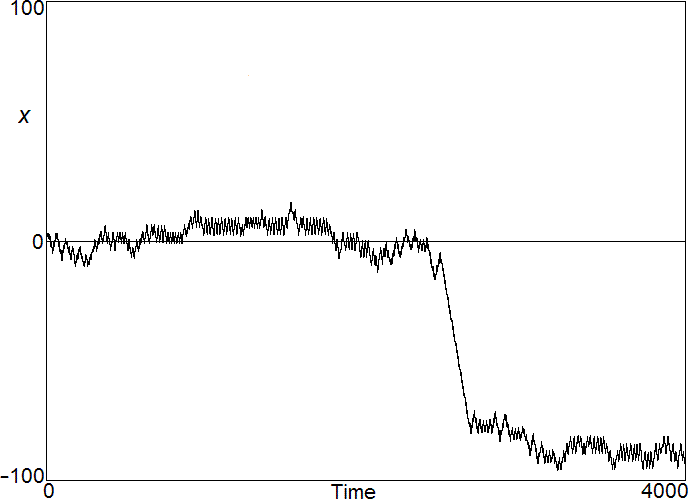Fig. 15. Standard deviation of 1.5 × 106 trajectories starting near the origin versus time.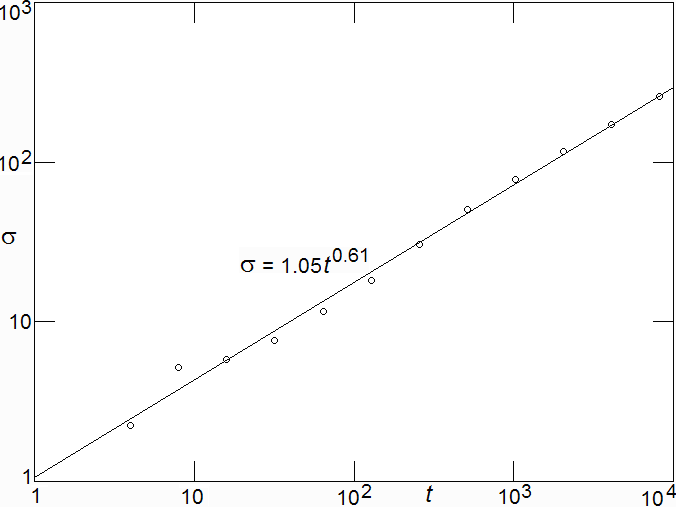Fig. 16. (a) Range versus time, and (b) autocorrelation function of dx/dt versus delay for an initial condition of (0.2, 0, 0).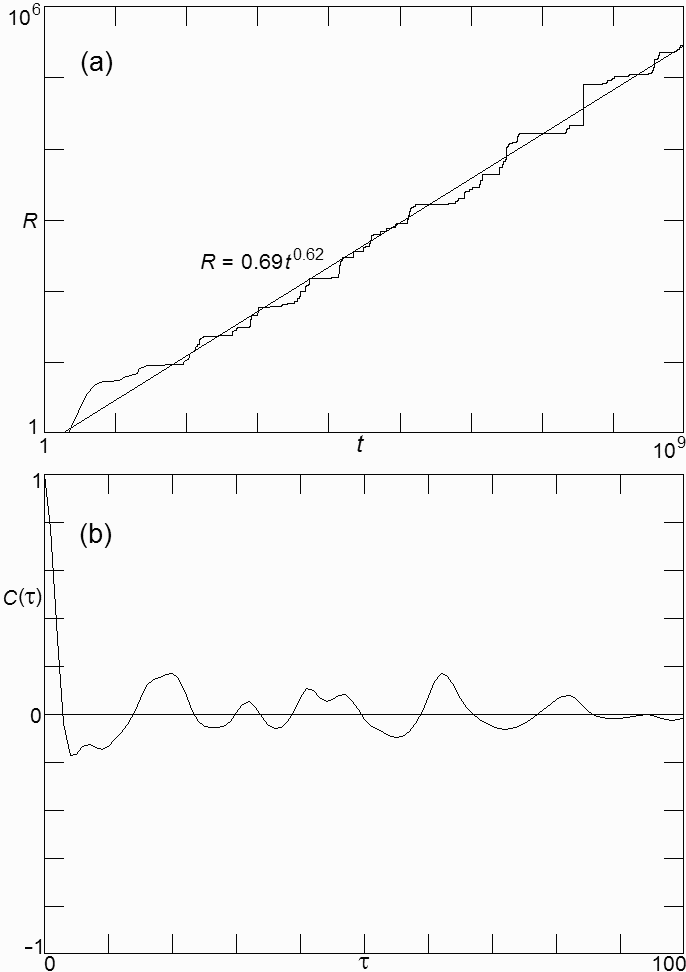Fig. 17. Allowable transitions for symbolic sequence.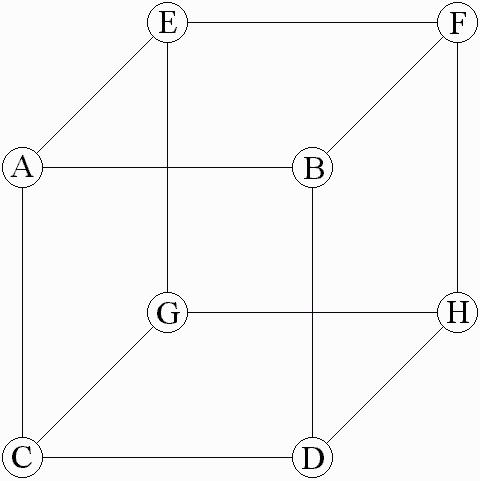Fig. 18. Iterated function system representation of symbolic dynamic.